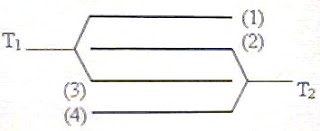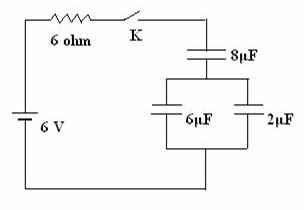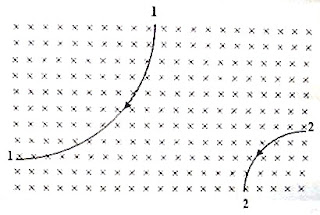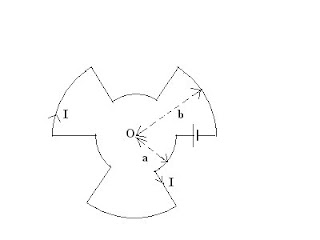## Pages

`“Life is like riding a bicycle.  To keep your balance you must keep moving.”–Albert Einstein`

## Saturday, May 22, 2010

### AP Physics C - Multiple Choice Practice Questions on Electrostatics

Multiple choice as well as free response practice questions on electrostatics were discussed earlier on this blog. You can access them by clicking on the label ‘electrostatics’ below this post or by trying a search using the search box provided on this page. Today we will discuss a few more multiple choice practice questions in this section:

(1) Ten identical capacitors, each of value 2 μF are connected in series and this series combination is connected across a regulated power supply of output 10 V. The energy stored in any one of the 2 μF capacitors is

(a) 1 J

(b) 2 J

(c) 5 J

(d) 10 J

(e) 100 J

The effective capacitance of the series combination is 2 μF/10 = 0.2 μF.

[If n capacitors each of value C are in series, the effective capacitance is C/n].

The energy of the series combination on charging with the 10 volt supply is ½ CV2 = ½×0.2×102 = 10 J.

Therefore, the energy of one capacitor = 10 J/10 = 1 J.

(2) Two equal positive charges of value 8 μC are placed in a region of space where there are no external fields. When a third charge q is placed at the mid point of the line joining the other two charges, the system is found to be in equilibrium. The third charge q must be

(a) 8 μC

(b) – 8 μC

(c) 4 μC

(d) – 4 μC

(e) – 2 μC

The third charge q must be negative so that the mutual repulsive force between the positive charges (Q each, let us say) is counteracted by the attractive force exerted by the negative charge.

Of course the above fact will come out from the expression for the net force on Q, which we will put equal to zero. Therefore we have

(1/4πε0)Q2/r2 + (1/4πε0)Qq /(r/2)2 = 0

Or, Q = – 4q so that q = – Q/4 = – 8 μC/4 = – 2 μC(3) The figure shows 4 identical parallel metallic plates [(1), (2), (3) and (4)] arranged with equal separation d between neighbouring plates. The surface area of one side of each plate is A and the medium between the plates is air. Alternate plates are joined to terminals T1 and T2 so that the system makes a parallel plate capacitor. Suppose there are n plates (instead of 4) in the system where n > 1 and may be odd or even. What is the capacitance of the system made of these n plates?

(a) 2n ε0A/d

(b) n ε0A/d

(c) (n – 1)ε0A/d

(d) (n + 1)ε0A/d

(e) n ε0A/2d

The lower surface of plate (1) and the upper surface of plate (2) makes a capacitor of capacitance ε0A/d. The lower surface of plate (2) and the upper surface of plate (3) makes another capacitor of capacitance ε0A/d. Similarly the lower surface of plate (3) and the upper surface of plate (4) makes a third capacitor of capacitance ε0A/d. These three capacitors are connected in parallel and the system give a total capacitance of 3ε0A/d.

If there are n plates, the effective capacitance C will be given by

(4) In the combination of capacitors shown in the adjoining figure, what is the effective capacitance between the terminals A and B?

(a) 20 C

(b) 11 C

(c) 8 C

(d) 6 C

(e) 3 C

On connecting a voltage source between the terminals A and B, the potential at the junction of the two capacitors of value 2C is the same as the potential at the junction of the two capacitors of value 4C.

[The capacitors need not necessarily be equal. It is enough that the ratios of capacitance are equal to balance the Wheatstone bridge]

The capacitors of value 8C is therefore connected between equi-potential points and it can be ignored (since it does not get charged). The network thus reduces to four capacitors with the series combination of 2C and 2C connected in parallel with the series combination of 4C and 4C. Therefore, the effective value is C + 2C = 3C.

(5) Point charges +2q, +2q and –q are placed at the vertices A, B and C respectively of an equilateral triangle ABC of side 2a. How much external work is to be done to move these charges so that the side of the equilateral triangle becomes a?

(a) (1/4πε0)(4q2/a)

(b) – (1/4πε0)(4q2/a)

(c) (1/4πε0)(2q2/a)

(d) – (1/4πε0)(2q2/a)

(e) Zero

The external work W to be done is given by

W = U2 U1 where U2 and U1 are respectively the final and initial electrostatic potential energies of the system.

Now, U2 = (1/4πε0)[(2q×2q)/a + 2q(–q)/a + (–q)2q/a] = 0

U1 = (1/4πε0)[(2q×2q)/2a + 2q(–q)/2a + (–q)2q/2a] = 0

Therefore, W = 0.(6) A conducting sphere of radius R is arranged concentrically inside a thin conducting spherical shell of radius 2R. The sphere carries a charge +q and the spherical shell carries a charge –Q. The potential difference between the sphere and the shell is

(a) (1/4πε0)(q/2R)

(b) (1/4πε0)(q/R Q/2R)

(c) (1/4πε0)(Q/2R)

(d) (1/4πε0) (qQ)/2R

(e) (1/4πε0) (qQ)/R

The potential V1 of the inner sphere is equal to the sum of the potentials due to its own charge q and the charge –Q on the shell:

V1 = (1/4πε0)(q/R) +(1/4πε0)(–Q/2R)

[Note that the potential due to the shell is constant everywhere inside it and is equal to (1/4πε0)(–Q/2R)].

The potential V2 of the outer shell is equal to the sum of the potentials due to its own charge –Q and the charge q on the sphere inside it. Since the total charge contributing to the potential of the shell is q Q, its net potential is given by

V2 = (1/4πε0)(qQ)/2R

The potential difference between the sphere and the shell is given by

V1 V2 = (1/4πε0)[(q/R) –(Q/2R) –(q/2R) +(Q/2R)]

Or, V1 V2 = (1/4πε0) [(q/R) –(q/2R)] = (1/4πε0)(q/2R)

* * * * * * * * * * * * * * * *

You can easily work out this problem if you note that the potential due to the charge on the shell appears in the net potential of the sphere inside it. Therefore, when you find the potential difference between the sphere and the shell, the contribution by the shell gets canceled and it is enough to find the potentials due to the charge on the sphere (sphere alone) at its surface and at distance 2R and find the difference:

Therefore the answer is simply (1/4πε0) (q/R) – (1/4πε0) (q/2R), which is equal to (1/4πε0)(q/2R).

Now suppose we have a conducting sphere of radius R1 carrying a charge Q1 arranged concentrically inside a thin conducting spherical shell of radius R2 carrying a charge Q2. The potential difference between the sphere and the shell is(1/4πε0) (Q1/R1) – (1/4πε0) (Q1/R2), which is equal to (1/4πε0)[(Q1/R1) – (Q1/R2)].

## Saturday, May 15, 2010

### AP Physics B & C - Multiple Choice Practice Questions on Electric Circuits Containing Resistors and Capacitors

Free response as well as multiple choice questions on direct current circuits containing resistors and capacitors are popular among question setters. Questions involving steady state are meant for AP physics B as well as AP physics C aspirants, where as questions involving RC transients are meant exclusively for AP physics C aspirants.

You will find questions in this section posted earlier on this site by performing a search using the search box on this page or by clicking on the label ‘direct current circuit’ below this page. Today we will discuss a few more practice questions in this section.

The following questions are meant for AP physics B as well as AP physics C aspirants:(1) In the circuit shown in the adjoining figure the capacitors are fully charged. What is the potential difference across the 2 μF capacitor?

(a) 0.5 V

(b) 1 V

(c) 2 V

(d) 3 V

(e) 4 V

The parallel combination of the 6 μF and 2 μF capacitors is equivalent to a single 8 μF capacitor. Therefore, the 6 V battery is (in effect) connected in series with two 8 μF capacitors so that there will be a potential difference of 3 V across each 8 μF capacitor. Since the parallel combination of the 6 μF and 2 μF capacitors makes the lower 8 μF capacitor, the potential difference across the 2 μF capacitor is 3 V.

[The potential difference across the 6 μF capacitor also is 3 V].

(2) In the circuit shown in question no.1 what is the charge on the lower plate of the 6 μF capacitor under steady state?

(a) 48 μC

(b) + 18 μC

(c) – 18 μC

(d) + 12μC

(e) – 12 μC

The lower plate of the 6 μF capacitor is connected to the negative terminal of the battery. The charge on the lower plate is therefore negative. As shown above (while answering question no.1), the potential difference across the 6 μF capacitor is 3 V. Therefore, the charge on the lower plate of the 6 μF capacitor is – (6 μF×3 V) = 18 μC [Remember Q = CV].

(3) In the circuit shown in question no.1 the battery has negligible internal resistance. What is the current through the 6 Ω resistor under steady state?

(a) 0.05 A

(b) 0.2 A

(c) 0.5 A

(d) 1 A

(e) zero

Under steady state the capacitors are fully charged and there is no charging current. The current through the 6 Ω resistor is therefore zero.(4) The circuit shown in the adjoining figure is almost the same as that shown in question no.1, but a key K is included. The internal resistance of the battery is negligible. Suppose the key K is open and the capacitors are initially uncharged. What is the current through the 6 Ω resistor at the moment the key K is closed (and the capacitors begin to get charged)?

(a) 0.05 A

(b) 0.5 A

(c) 1 A

(d) 2 A

(e) zero

At the moment the key is closed, there are no charges on the capacitors to oppose the flow of charges delivered by the battery. The capacitors therefore allow the passage of the transients with ease and the only opposition to the flow of the charges in the circuit is that offered by the resistor. The initial current through the 6 Ω resistor is therefore 6 V/6 Ω = 1 A.

The following questions are for AP Physics C aspirants:

(5) Refer to the circuit shown in question no. 4. Initially the key K is open and the capacitors are uncharged. After closing the key, what time must be elapsed for the current through the 6 Ω resistor to become 1/e ampere (where ‘e’ is the base of natural logarithms)?

(a) 24 μs

(b) 48 μs

(c) 96 μs

(d) 0.01 s

(e) 0.1 s

The initial charging current I0 through the 6 Ω resistor is given by

I0 = V/R = 6 V/6 Ω = 1 A.

The time constant of an RC circuit is the time required for the charging current to reduce from its initial value I0 to (1/e)I0. If you remember this fact, you can immediately write down the answer as RC since I0 = 1 A in the present case. So the answer is 6 Ω×4 μF = 24 μs.

[Usually the time constant of an RC circuit is defined as the time required for the charge on the capacitor (or voltage across the capacitor) to grow from zero to (1– 1/e) times the final steady value. The growth of charge Q is exponential and is given by

Q = Q0 (1 – e–t/RC) where Q0 is the final steady charge.

The charging current I at time t after closing the key K is given by

I = dQ/dt = (Q0/RC)e–t/RC = (CV/RC)e–t/RC = (V/R)e–t/RC = I0e–t/RC.

Therefore, I = (1/e)I0 when t = RC](6) In the circuit shown in the adjoining figure the capacitor C is initially charged to have a potential difference V0 volt across it. The key K is initially open. When the key K is closed (at zero time), the potential difference across the capacitor becomes 0.5 V0 volt after a time t . What is the value of t ?

(a) 2 RC

(b) 0.5RC

(c) RC/ln2

(d) RC ln2

(e) RC

The potential difference V after time t is given by

V = V0 e–t/RC where ‘e’ is the base of natural logarithms.

Therefore we have 0.5 V0 = V0 e–t’/RC.

Or, et’/RC = 1/0.5 = 2 from which t/RC = ln2

This gives t’ = RC ln2.

## Thursday, May 6, 2010

### AP Physics Exam – Final Preparation - Make Full Use of Your Time

You have very limited time left for your preparation for the AP Physics Exam. I have often wondered why the number of visitors to this blog is unusually large on the day previous to the exam day. Do not postpone things related to the exam to the final hours of your preparation.

While answering multiple choice questions, you are at liberty to try all shortcuts and your prime concern should be to save as much time as possible, of course, without committing mistakes by carelessness. Free response questions are to be answered taking into account the guidelines prescribed by the College Board.

You should have a clear idea about what you can (and should) take to the examination hall. Of course you should have an equally clear idea about what you cannot take to the examination hall. The College Board gives very clear instructions in this regard and you must certainly go through them. Visit the College Board web site

http://www.collegeboard.com/student/testing/ap/exday.html

Control your exam stress and take care of your health. You will find a short post in this context here.

## Sunday, May 2, 2010

### AP Physics B & C – Magnetic Fields – Multiple Choice Practice Questions

Questions involving magnetic fields were discussed on many occasions on this blog. You can access them by trying a search for ‘magnetic field’ using the search box provided on this page or by clicking on the label ‘magnetic field’ below this post.
The following multiple choice practice questions involving magnetic fields will be worth noting even if you find very limited time for your final preparation for the fast approaching AP Physics B as well as AP Physics C examination:(1) The adjoining figure shows the paths of two particles (1 and 2) carrying charges of the same magnitude, when projected, with the same speed, at right angles to a uniform magnetic field. The magnetic field is directed normally into the plane of the figure. Pick out the correct statement from the following:

(a) Particle 1 is negatively charged, particle 2 is positively charged and particle 2 is heavier than particle 1.

(b) Particle 1 is negatively charged, particle 2 is positively charged and particle 1 is heavier than particle 2.

(c) Particle 1 and particle 2 are positively charged and particle 2 is heavier than particle 1.

(d) Particle 1 and particle 2 are negatively charged and particle 2 is heavier than particle 1.

(e) Particle 1 is positively charged, particle 2 is negatively charged and particle 1 is heavier than particle 2.

Since the amounts of charges are equal, the magnetic forces in the uniform field are of equal magnitude. So the radius of curvature of the heavier particle is greater, which is the case with particle 1.

[We have mv2/r = qvB, on equating the centripetal force to the magnetic force so that the radius of the path is given by

r = mv/qB

Since v, q and B are the same, r must be greater for the heavier particle].

On applying Fleming’s left hand rule (motor rule) to particle 2 we obtain the sense of bending as expected for a positively charged particle.

[Hold your fore-finger along the direction of the magnetic field and the thumb along the direction of bending (direction of magnetic force). If the direction of motion of the particle is representable by the middle finger, the particle is positively charged. Otherwise, it is negatively charged].

Particle 1 is found to be negatively charged on applying Fleming’s left hand rule. The correct option is (b).

(2) A proton is moving in a static uniform magnetic field. There are no other fields. Which one of the following statements is false?

(a) The kinetic energy of the proton remains unchanged

(b) The magnetic field can exert a force on the proton only if it is in motion.

(c) The magnetic field changes the velocity of the proton.

(d) The magnetic field reduces the speed of the proton

(e) The magnetic field does not change the kinetic energy of the particle.

Since the magnetic force on the proton is always at right angles to its displacement, no work is done by the magnetic field. It can just deflect the proton, thereby changing the direction of its velocity, without altering its magnitude (speed).

(3) Two long straight conductors C1 and C2 carrying the same current I flowing in the positive z-direction are arranged parallel to the z-axis of a right handed Cartesian coordinate system. The conductors are at the same distance s from the z-axis, as shown in the figure. What is the magnitude of the resultant magnetic flux density at the origin?

(a) μ0I/πs

(b) μ0I/2πs

(c) √2 (μ0I/πs)

(d) (1/√2)(μ0I/πs)

(e) zero

Conductor C1 and C2 carrying the current I produce magnetic fields of the same magnitude (B = μ0I/2πs) at the origin O. These fields are at right angles to each other and hence the resultant field at the origin is [(μ0I/2πs)2 + (μ0I/2πs)2 ]1/2 = √2 0I/2πs) = (1/√2)(μ0I/πs), as given in option (c).

[Note that the field at the origin due to C1 is along the positive y-direction where as the field at the origin due to C2 is along the positive x-direction. The resultant field is inclined at 45º with the positive x-direction and the positive y-direction].

(4) A time varying magnetic field is applied in a region of space where a stationary electron and a moving electron are present. Ignore the interaction between the electrons (as they are sufficiently farther apart). Then

(a) the stationary electron and the moving electron are unaffected.

(b) the stationary electron and the moving electron experience forces.

(c) the stationary electron is unaffected where as the moving electron experiences forces.

(d) the moving electron is unaffected where as the stationary electron experiences forces.

(e) the moving electron comes to rest where as the stationary electron starts moving.

The correct option is (b) since a changing magnetic field will produce an induced emf and the stationary electron and the moving electron will experience forces.

The following question is meant for AP Physics C aspirants; but AP Physics B aspirants too can try to solve it:(5) A conductor is bent in the form shown in the adjoining figure so that there are six straight radial portions of equal length and six concentric arcs of circles of radii ‘a’ and ‘b’, subtending the same angle at the common centre O. A battery connected in the circuit drives a steady current ‘I’ through the circuit. What is the magnitude of the magnetic flux density at the centre O?

(a) μ0I(a+b)/4ab

(a) μ0I(a+b)/3ab

(c) μ0I(a+b)/2ab

(d) μ0I(a+b)/ab

(e) Zero

The straight radial portions will not produce any magnetic field at the centre O. The field at the centre is produced by three identical arcs of a circle of radius ‘a’ and three identical arcs of a circle of radius ‘b’. The three smaller arcs will make a semicircle of radius ‘a’ and the three larger arcs will make a semicircle of radius ‘b’. The magnetic fields produced by them are in the same direction (acting normally into the plane of the figure) and hence they add up to produce a resultant field at the centre O.

Therefore, the magnetic field B at the centre O is given by

B = ½ × μ0I/2a + ½ × μ0I/2b

[The factor ½ appears because the fields are due to semicircular currents].

Thus B = (μ0I/4)(1/a + 1/b) = μ0I(a+b)/4ab

[Suppose we have a circuit of the form shown below: